## Tuesday, August 8, 2023

### Will I draw an epidemic card in Pandemic? (and more)

When an epidemic card is drawn in the cooperative game Pandemic, it ramps up the difficulty of the game. Knowing when this occurs, or when it could occur, is useful to players to achieve victory. Here, one question ends up leading to additional questions. As we know more about a problem, the horizon of our ignorance often expands, rather than contracts. However, by taking what we learn in answering one question we're often better positioned to answer the next one.

## Pandemic setup and epidemic cards

Pandemic has an unusual setup procedure for the player deck. For standard difficulty with 4 players there are 48 city cards, 5 event cards, and 5 epidemic cards summing to 58 total cards. However, $2\cdot 4=8$ of these cards are dealt initially to the players, 2 cards to each of the 4 players. This results in there being $48+5+5-2\cdot 4=50$ cards in the player deck at the start of the game. However, these are not all shuffled together. Each of the 5 epidemic cards are separated with an equal number of other cards to form 5 piles of 10 cards per pile ($50/5=10$). Each pile is shuffled separately and then stacked on top of each other.

There is a separate infection deck containing 48 city cards that will come to our attention later. Cards are drawn from the infection deck each turn to determine where disease spreads in the game.

When an epidemic card is drawn three things occur. First, the number of cards drawn from the infection deck each turn may increase. Second, a new city is infected by drawing from the bottom of the infection deck (guaranteeing it has not been drawn before) with three cubes. Third, the infection deck discard pile is shuffled together and put on top of the deck. This means currently and previously infected cities get drawn again. The next step in the game is to draw from the infection deck, where any cards drawn for a city already containing three disease cubes triggers an outbreak. This causes more disease cubes to be placed in neighboring cities. Among other loss conditions, players lose if there are too many cubes placed or too many outbreaks.

## Will I draw an epidemic card in Pandemic?

On each turn, 2 player cards are drawn. The probability of drawing an epidemic card on any particular turn, without knowing anything else is $1/5=0.2$. There are at least two ways to think about it and it's instructive to consider both. The probability that any single card drawn being an epidemic card is $1/10=0.1$, see Fig. 1.Figure 1: 10 cards from one pile

This would be the same even if we didn't make special preparations to the deck, though perhaps we'd think of the calculation as $5/50=0.1$ as 5 of the 50 card in the initial deck are epidemic cards. Since players draw 2 cards per turn, each initial pile of 10 cards is drawn across exactly 5 turns, as depicted in Fig. 2.  (While the piles are combined at the beginning of the game, I'll refer to the current pile as the set of cards that were in a pile before they were stacked to make the whole deck.)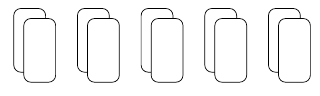Figure 2: Drawing each 10-card pile over 5 turns

Exactly 1 of those 5 pairs of cards contains an epidemic card. Thus, the probability is $1/5=0.2$. However, we should be careful about thinking this way. It's tempting to think of this probability as the sum of the probabilities of drawing an epidemic card for each of the two cards drawn on the turn. This only works here because drawing an epidemic card as the first card in a turn and drawing one as the second card are not independent events. The probability of drawing an epidemic card on a given turn is equal to the sum of the probability of drawing it as the first card plus the probability of drawing it as the second card, because these events are disjoint, or mutually exclusive, events.  (Note that this is not always true in Pandemic. For some player counts and difficulty levels, there can be an uneven number of cards in some of the player deck piles at the beginning of the game. In such a case, if the last card of one pile and the first card of the next pile are both epidemic cards, then two epidemic cards can be drawn on a single turn.)

\begin{align} P(\text{E during turn}) = P(\text{E as first card}) + P(\text{E as second card}) \end{align}

(I've abbreviated "draw epidemic" as "E" to shorten the equations.)

From here, we could complete the computation, as we know the probability of any card being an epidemic card in isolation is 0.1. However, we want to describe these events in a way that is easy to construct with probability that doesn't feel so hand wavy. Or, at least highlights the difference compared to when the events are not disjoint. The only way to draw an epidemic card as the second card is if we didn't draw it on the first, thus that event is equivalent to not drawing an epidemic card and then drawing an epidemic card. Similarly, the only way to draw an epidemic card as the first card is to draw an epidemic card and then not draw one.

\begin{align} P(\text{E during turn}) &= P(\text{E then not E}) + P(\text{not E then E}) \end{align}

Let's rewrite this in terms of a logical and, or the intersection of events.

\begin{align} P(\text{E then not E}) &= P(\text{first E} \cap \text{second no E}) \\ P(\text{not E then E}) &= P(\text{first no E} \cap \text{second E}) \end{align}

We can break this up into independent pieces using the multiplication rule, $P(A \cap B) = P(A|B) \cdot P(B)$, though we'll turn the order around a little bit in terms of $A$ and $B$ to write them in the order of occurrence. $P(A|B)$ is a conditional probability, the probability of $A$ given that $B$ occurs. This allows us to chain events together, as shown below.

\begin{align} P(\text{first E} \cap \text{second no E}) &= P(\text{first E}) \cdot P( \text{second no E}|\text{first E}) \\ P(\text{first no E} \cap \text{second E}) &= P(\text{first no E}) \cdot P( \text{second E}|\text{first no E}) \end{align}

Now, let's plug in the numbers. For the first draw, the probabilities are $1/10$ and $9/10$, as either 1 or 9 of the 10 cards are relevant. For the second draw under these conditions there are only 9 cards remaining, and either 9 or 1 of them are of interest.\begin{align} P(\text{first E}) &= \frac{1}{10} \\ P( \text{second no E}|\text{first E}) &= \frac{9}{9} \\ P(\text{first no E}) &= \frac{9}{10} \\ P( \text{second E}|\text{first no E}) &= \frac{1}{9} \end{align}

We can combine our equations to get the following.

\begin{multline} P(\text{E during turn}) = P(\text{first E}) \cdot P( \text{second no E}|\text{first E}) \\ + P(\text{first no E}) \cdot P( \text{second E}|\text{first no E}) \end{multline}

Now we plug in the numbers.

\begin{align} P(\text{E during turn}) &= \frac{1}{10} \cdot \frac{9}{9} + \frac{9}{10}\cdot\frac{1}{9}\\ &= \frac{1}{10} + \frac{1}{10}\\ &= 0.2 \end{align}

We can contrast this with rolling dice, to see the care we should take when adding probabilities. Consider the probability of rolling a 3 on 2d10. (Two 10-sided dice numbered 1 through 10 are here referred to as 2d10.)  This could either be for exactly one 3 or at least one 3. The probability of getting a 3 on a single d10 is $1/10=0.1$, similar to our Pandemic question. However, since each roll is independent, the probability of one roll does not affect the next. Thus, the probability of getting exactly one 3 is $\frac{1}{10}\cdot\frac{9}{10} + \frac{9}{10}\cdot\frac{1}{10} = 0.18$, which is somewhat less than the 0.2 for the Pandemic scenario, using a similar calculation form. The probability of getting at least one 3 is computed as $1-P(\text{no 3s}) = 1 - \left (\frac{9}{10}\right )^2 = 0.19$, a higher probability, but still less than 0.2.

It is close to 0.2, though. Note the equation we used above, we can generalize to $1 - (1-p)^2$, where $p$ is the probability of occurring in one of the two trials. This equations expands to,

\begin{align} 1 - (1-p)^2 = 1 - (1 - 2p + p^2) = 2p - p^2. \end{align}

When $p$ is small, $p^2 \ll 2p$, so $2p$ is a good approximation.

The distinction here between the behavior observed with dice and cards is ripe for reflection. (It's not so much dice vs. cards, but rather independent vs. dependent. If drawing cards with replacement, the same probabilities hold as with dice.)  For the probability of rolling at least one 3, note that the second roll only increases the probability if the first roll was not a 3. This means much of the time, indeed with probability $\frac{1}{10} \cdot \frac{1}{10} = 0.01$, the second roll does no good in terms of counting as rolling a 3. This is an additional case that does not show up for our Pandemic question. In the scenario considered, it is not possible for both cards drawn on one turn to be epidemic cards, but it is possible to get two 3s when rolling 2d10.

Note also that the piles of 10 cards is important to the calculation. If instead there were simply 5 epidemic cards somewhere in the 50 card deck, then the probability of drawing an epidemic card as the first card in a turn is unchanged, but the second card is different and depends on whether the first card is an epidemic card or not. Here we'll use E$_{50}$ to denote drawing an epidemic card with a fully shuffled player deck.

\begin{align} P(\text{first E}_{50}) &= \frac{5}{50} \\ P( \text{second no E}_{50}|\text{first E}_{50}) &= \frac{45}{49} \\ P(\text{first no E}_{50}) &= \frac{45}{50} \\ P( \text{second E}_{50}|\text{first no E}_{50}) &= \frac{5}{49} \end{align}

Since here we can also get two epidemic cards, we have an additional probability of interest.

\begin{align} P( \text{second E}_{50}|\text{first E}_{50}) &= \frac{4}{49} \end{align}

Putting this together, we get the following.

\begin{multline} P(\text{E$_{50}$ during turn}) = P(\text{first E}_{50}) \cdot P( \text{second no E}_{50}|\text{first E}_{50}) \\ + P(\text{first E}_{50}) \cdot P( \text{second E}_{50}|\text{first E}_{50}) \\ + P(\text{first no E}_{50}) \cdot P( \text{second E}_{50}|\text{first no E}_{50}) \end{multline}

This simplifies, as it doesn't matter what we draw as a second card if the first is an epidemic card. Put another way,

\begin{align} P( \text{second no E}_{50}|\text{first E}_{50})+ P( \text{second E}_{50}|\text{first E}_{50}) = 1. \end{align}

Plugging that in, we get the following.

\begin{align} P(\text{E$_{50}$ during turn}) &= P(\text{first E}_{50}) + P(\text{first no E}_{50}) \cdot P( \text{second E}_{50}|\text{first no E}_{50}) \\ &=\frac{5}{50} + \frac{45}{50} \cdot \frac{5}{49} \\ &\approx 0.192 \end{align}

An alternative way of thinking of the earliest computation is that we are separating the piles of 10 cards into two groups: the 2 cards we draw this turn and the 8 cards we draw on the other four turns. Thus the probability of drawing the one epidemic card is 2 cards out of 10 total, or 0.2. We can think of this construction as choosing where to place the epidemic card. There are $\binom{2}{1}=2$ ways to put it in the 2 cards of interest and $\binom{10}{1}=10$ total ways to put it in a particular pile.

## What if we're in the middle of the game?

The single probability that we calculated is limited, though, as it doesn't match the amount of information that we have for much of the game. Once we draw cards, we know more about some of the remaining turns. We can compute the conditional probabilities, the probabilities given the knowledge we have from either drawing or not drawing epidemic cards on previous turns. For example, after not drawing an epidemic card in the first turn, the probability of drawing an epidemic card on the second turn increases, as now there are only 8 cards in the current pile, or equivalently 4 turns until the current pile is exhausted. This is similar to when we looked at the length of a game of High Society, except here we repeat through multiple piles. The conditional probability can also drop to zero. If you draw an epidemic card on the first turn, you know that you won't for the next 4 turns.

The probability of drawing an epidemic card on the second turn given that we did not on the first turn is $2/8=0.25$. Of course, if the probability of drawing an epidemic card on the second turn given that we did draw one of the first turn is 0. We can extend this for the third turn ($2/6=1/3$ and $0$), fourth turn ($2/4=0.5$ and $0$) and fifth turn ($2/2=1$ and $0$). On the sixth turn we draw from the second pile, so the previous set of probabilities repeat. We can write the probability, $p_\text{not}[n]$, of drawing an epidemic card on turn $n$ given that we have not drawn one from the current piles as,

\begin{align} p_\text{not}[n] = \frac{2}{10 - 2\cdot ( (n-1)\mod 5 )}, \end{align}

where $a \mod b$, or $a$ modulo $b$, is the remainder after dividing $a$ by $b$. This forms the desired repeating pattern. The above equation could be rewritten in a simplified form, but it is based on the number of cards, which are the physical counts that give rise to the probabilities. The $(n-1) \mod 5$ term represents the number of turns where cards were already drawn from the current pile. These probabilities are plotted in Fig. 3 along with the probability given we already drew the current epidemic card (if possible) as well as the unconditional probability.  (The probability is set to 0.2 for the first turn of each pile to emphasize that the probability here is always 0.2.)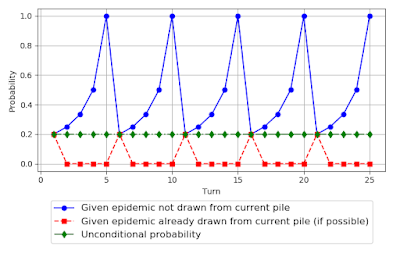Figure 3: Probability of drawing an epidemic card in Pandemic conditioned on whether one was drawn from the current pile

The plots make the periodic nature quite apparent, even aside from the logical necessity given the setup.

## How many turns are there between epidemic cards?

This leads us to question the distribution of the number of turns between epidemic cards, as this can impact the game in notable ways. Do we get new heavily infected cities back to back, or a long gap to take care of the current situation. To find the distribution, we construct five random variables, $X_1$ through $X_5$, for the five turns on which the epidemic cards are drawn, and then four additional random variables, $Y_2$ through $Y_5$, that represent the differences between the first five. $Y_k$ is the number of turns since the previous epidemic card after the $k$-th epidemic card.

\begin{align} Y_k = X_{k} - X_{k-1} \end{align}

The first five are uniform random variables, as each possible value occurs with equal probabilities. That is, we know the first epidemic card has a probability 0.2 of being drawn in each of the first five turns. Thus, we can write the probability mass function (pmf) of the random variable $X_1$, the turn in which the first epidemic card is drawn, as follows.

\begin{align} p_{X_1}(x) = \begin{cases} 0.2 & x \in \{1, 2, 3, 4, 5\} \\ 0 & \text{else} \end{cases} \end{align}

The pmfs of the random variables for the other turns in which an epidemic card is drawn are similar.

\begin{align} p_{X_k}(x) = \begin{cases} 0.2 & x \in [5 (k-1)+1, 5 k] \\ 0 & \text{else} \end{cases} \end{align}

These pmfs are plotted in Fig. 4.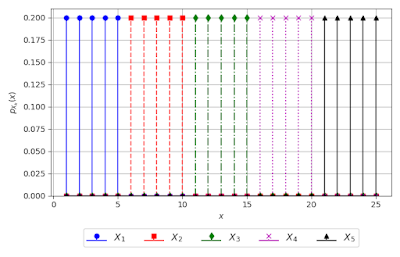Figure 4: Probability mass functions of the turn in which each epidemic card is drawn

When discussing D&D ability scores, we introduced convolution as a mathematical operation to determine the pmf of the sum of two random variables from their pmfs.  (Convolutions have other uses as well.) We can use convolution here to find the pmfs of the differences $Y_k$. While $Y_k$ are equal to the difference of known random variables, rather than the sum, the same principle applies. We can see this by first transforming $X_k$ into negative versions, $X'_k = -X_k$. We know the pmfs.

\begin{align} p_{X'_k}(x) &= p_{X_k}(-x) \\ &= \begin{cases} 0.2 & x \in [-5 k, -5 (k-1)-1] \\ 0 & \text{else} \end{cases} \end{align}

With this, we can write $Y_k$ in terms of the sum of random variables with known pmfs.

\begin{align} Y_k = X_{k} + X'_{k-1} \end{align}

And thus state the pmfs in terms of convolution.

\begin{align} p_{Y_k}(y) = p_{X_{k}}(y) * p_{X'_{k-1}}(y) \end{align}

Since $X_k$ and $X'_k$ have uniform distributions that have a rectangular shape when considered over all $x \in \mathbb{Z}$ (the set of all integers), the differences $Y_k$ have a triangular distribution from convolution. This is the same as how the sum of 2d6 has a triangular distribution stemming from the rectangular pmfs of each individual d6. We could go through the motions, but this is a general truth when the rectangular pmfs have equal length. That is, when they are non-zero for a range of the same size. Otherwise, there is a flat portion in the middle. It is worth considering something like 1d6+1d8 to see this effect. If we take the shape as given, then we can find the relevant constants.

We can argue from the situation the maximum and minimum possible values of $Y_k$. It has a minimum value of 1, which occurs when the epidemic card in one pile is drawn as late as possible, while the one in the next pile is drawn as early as possible. It has a maximum value equal to $10-1=9$. Here I'm looking at the latest possible turn the second epidemic card can be drawn (turn 10) and the earliest the first can be (turn 1). It is the same for all $Y_k$. If we're familiar with convolution, we'd also know that it has this property that the length of of the convolution of two finite sequences with lengths $n$ and $m$ is $n+m-1$, which in this case is $5+5-1=9$. This necessarily follows from the convolution definition.

\begin{align} p_{X_{k}}(y) * p_{X'_{k-1}}(y) &= \sum_{n=-\infty}^\infty p_{X_{k}}(y-n) \cdot p_{X'_{k-1}}(n) \\ &= \sum_{n=-\infty}^\infty p_{X_{k}}(n) \cdot p_{X'_{k-1}}(y-n) \label{eq:pandemic_convolve} \end{align}

There is some symmetry in that the two random variables being added have equal values over the same range. Combined with the symmetry of convolution itself, that $f(x) * g(x) = g(x) * f(x)$, the resulting convolution must be symmetric about some point. This must be the mid-point between 1 and 9, which is 5. This makes a lot of sense, since each pile is five turns of card draws it is most likely that that is how far apart they are. We could find the probabilities by combining the known shape with the fact that the pmf must sum to 1 to be valid, but it's easier to just use a computer to convolve some vectors of copies of 0.2 or look at.

We can use the visual method of computing convolutions, illustrated in Fig. 5 for $p_{Y_2}(y) = p_{X_{2}}(y) * p_{X'_{1}}(y)$. Following an earlier equation, we plot $p_{X_2}(n)$, a version of $p_{X'_1}(n)$ that is horizontally flipped and shifted by $y$, their product, and the running sum, across several values of $y$. To save space, not all values of $y$ are plotted.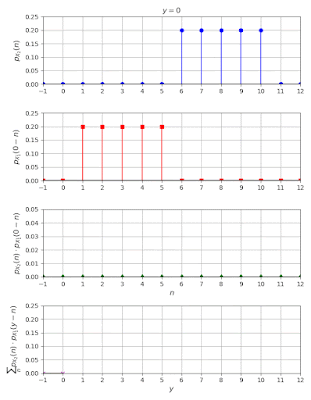Figure 5: Illustrating convolution to find pmf of $Y_k$

More numerically, we can look at the peak value of $p_{Y_k}(y)$. This occurs at $y=5$ and corresponds to when the flipped and shifted $p_{X_{k}}(n)$ is exactly on top of $p_{X'_{k-1}}(n)$. Thus, the convolution yields $p_{Y_k}(5) = 5 \cdot 0.2^2 = 0.2$. This is enough to construct the pmf shown in Fig. 6 with the arguments made earlier.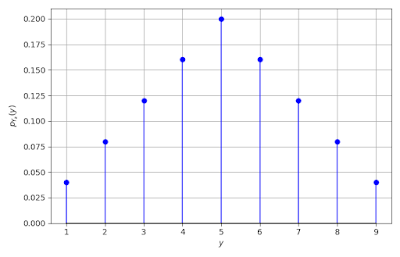Figure 6: Probability mass function of the number of turns from one epidemic card to the next

While $X_k$ are independent, as shuffling one pile does not affect the next, $Y_k$ are not independent. The dependence is quite clear as $Y_2 = X_2 - X_1$ and $Y_3 = X_3 - X_2$. Both depend on $X_2$. If the second epidemic card come early, that means the first interval is short while the second interval is long. We could capture this dependence by looking at the joint distribution of all $Y_k$. While some pairs are independent, for example the first interval, $Y_2$, and the last, $Y_5$, they all connect via other intervals. However, this looks to be a tedious and uninspiring calculation. We know the main gist of the dependence and leave it at that for now.

## What is the probability of drawing the newly infected city after an epidemic card?

One of the things that can happen after an epidemic card is drawn is that the newly infected city is drawn from the infection deck. The probability of this occurring is a function of how many cards are in the discard pile of the infection deck, which in turn depends on how many turns are between epidemic cards (or since the beginning of the game).

First let's ask an easier question, let's look at after just the first epidemic card. We start with 9 cards in the infection deck discard pile. Then we get 2 more per turn until the epidemic card is drawn. This means we get 0, 2, 4, 6, or 8 more infection cards (epidemic cards are in the player deck, which is drawn from before drawing from the infection deck). One more card is added from the epidemic card itself (from the bottom of the infection deck). All these cards get shuffled and placed atop the infection deck. Thus, there are 10 to 18 cards in which the newly infected city card is shuffled. After the first epidemic card, we're still drawing two infection cards per turn, so the probability of drawing the newly infected city is 2 divided by the number of cards that were shuffled and put on top. This repeats the same concept as we used to find the probability of drawing an epidemic card in the first place, that we're drawing from a deck without replacement. In the equations, I'll abbreviate "outbreak in newly infected city immediately after the first epidemic card" as "O from E1".

\begin{align} P(\text{O from E1} | \text{E on turn 1}) &= \frac{2}{10} \\ P(\text{O from E1} | \text{E on turn 2}) &= \frac{2}{12} \\ P(\text{O from E1} | \text{E on turn 3}) &= \frac{2}{14} \\ P(\text{O from E1} | \text{E on turn 4}) &= \frac{2}{16} \\ P(\text{O from E1} | \text{E on turn 5}) &= \frac{2}{18} \end{align}

As we found before, the 5 possibilities are equally likely, so the total probability is as follows.

\begin{align} P(\text{O from E1}) &= \frac{1}{5}\cdot\frac{2}{10} + \frac{1}{5}\cdot\frac{2}{12} + \frac{1}{5}\cdot\frac{2}{14} + \frac{1}{5}\cdot\frac{2}{16} + \frac{1}{5}\cdot\frac{2}{18} \\ &\approx 0.149 \end{align}

This is the probability of this happening before we know anything about how the game goes. Obviously, once the epidemic card is actually drawn, then the probability is $\frac{1}{10}$ or $\frac{1}{14}$ or one of the other possibilities depending on how many cards are actually in the infection deck discard pile. This probability speaks to how likely it is that the deck is stacked against us.

Now, let's look at the probability of this happening after the second epidemic card. Here, we can use the distribution of the number of turns from one epidemic card to the next, in this case $Y_1$. Through this time, we're still drawing 2 infection cards per turn, so the number of cards in the infection deck discard pile is $1+2Y_2$, one from the epidemic card, and two for each turn since the last epidemic card. Using the same logic as above, we find the probability of drawing the newly infected city conditioned on the value of $Y_2$.

\begin{align} P(\text{O from E2} | Y_2 = y) &= \frac{2}{1+2y} \end{align}

To get the total probability, we need to sum over all possible $Y_2$.

\begin{align} P(\text{O from E2}) &= \sum_{y=1}^9 \frac{2}{1+2y}\cdot p_{Y_2}(y) \\ &\approx 0.219 \end{align}

Getting to the third epidemic card gets interesting, because now we start to draw more infection cards per turn. We'll define $r_k$ to be the rate of infection after the $k$-th epidemic card is drawn, with $r_0$ being the rate at the start of the game. These rates are shown in Table 1.

\begin{array}{ccccccc} r_0 & r_1  & r_2  & r_3  & r_4  & r_5  & r_6 \\ \hline 2 & 2 & 2 & 3 & 3 & 4 & 4 \end{array} Table 1: Infection rate, $r_k$, after the $k$-th epidemic card

Note that this includes $r_6$, which is only relevant for the heroic difficulty level. In the third epidemic card case, we still were drawing 2 infection cards per turn until the epidemic card, so the three infection cards being drawn only affects the probability of drawing the newly infected city, not how many cards there are.

\begin{align} P(\text{O from E3}) &= \sum_{y=1}^9 \frac{3}{1+2y}\cdot p_{Y_3}(y) \\ &= \frac{3}{2} \cdot P(\text{O from E2})\\ &=0.329 \end{align}

Note that the pmfs of all four differences rare the same, so $p_{Y_2}(y) = p_{Y_3}(y)$.

For subsequent epidemic cards, though, the higher rate of infection card draw changes the number of cards in the infection card discard pile in which the newly infected city is shuffled. For example, for the fourth epidemic card, there are now $1+3Y_4$ cards, three for each of the $Y_4$ turns from the third epidemic card to the fourth. In general, there are $1+r_{k-1} \cdot Y_k$ cards in the infection deck discard pile after the $k$-th epidemic card. Thus, we can generalize our equation.

\begin{align} P(\text{O from E$k$}) &= \sum_{y=1}^9 \frac{r_k}{1+r_{k-1}\cdot y}\cdot p_{Y_k}(y) \qquad k \geq 2 \label{eq:p_outbreak_k} \end{align}

One thing that's worth checking is that we don't create nonsensical probabilities. In this case, I'm concerned that our generalized formula could erroneously give probabilities above 1 in the $\frac{r_k}{1+r_{k-1}\cdot y}$ term for small $y$. However, since $y \geq 1$ and $r_k \leq r_{k-1} + 1$, then this correctly gives a probability of 1 when epidemic cards are drawn back to back and the infection rate increases by one.

\begin{array}{ccc} k & r_k & P(\text{O from E$k$}) \\ \hline 1 & 2 & 0.149 \\ 2 & 2 & 0.219\\ 3 & 3 & 0.329\\ 4 & 3 & 0.230\\ 5 & 4 & 0.307 \end{array} Table 2: Probability of outbreaking in newly infected city, after the $k$-th epidemic card

The computed approximate probabilities are listed in Table 2. It is interesting to see how these probabilities change over the course of the game. The first we would expect to be lower, as the beginning of the game is seeded with an additional 9 cards, while after the later epidemic cards it is possible to have only a few cards.  We also see the probabilities jump when the infection rate increases. This makes sense, as the number of cards drawn immediately after an epidemic card is relatively high. What may not be clear is why $P(\text{O from E$4$})$ is higher than $P(\text{O from E$2$})$ while $P(\text{O from E$5$})$ is lower than $P(\text{O from E$3$})$. The effect of more cards being drawn overall has a different effect when $r_k = r_{k-1}$ compared to when $r_k = r_{k-1}+1$. Looking at the denominator $1+r_{k-1}\cdot y$ again indicates that as $r_{k-1}$ increases, the constant 1 card from the epidemic card has a smaller effect, making the probability increase. That explains why $P(\text{O from E$4$})$ is higher than $P(\text{O from E$2$})$. In the other direction, as $r_{k-1}$ increases, having $r_k = r_{k-1} + 1$ in the numerator makes a smaller increase over $r_k = r_{k-1}$. In the limit there is no increase.

\begin{align} \lim_{r_{k-1} \to \infty} \frac{r_{k-1}+1}{1+r_{k-1} \cdot y} = \frac{1}{y} \end{align}

That explains why $P(\text{O from E$5$})$ is lower than $P(\text{O from E$3$})$.

Looking at the probabilities of getting an outbreak in the newly infected city after each epidemic card, $P(\text{O from E$k$})$, it is clear that the total probability is not just the sum, as that would be well above 1, which is the maximum valid value for any probability. This indicates that these probabilities are not independent. Indeed they are not, as they are based on the number of turns from one epidemic card to the next, which themselves are not independent. If we had the joint probability distribution then we could calculate the total probability. That is not simple to come by here, so we'll take two approaches to get what we want.

One approach is to set aside the probability of getting such an outbreak and rather look at the expected number of such outbreaks. This simplifies our calculations, because when taking the expected value of the sum of some random variables it is equal to the sum of the expected values of those random variables, even if the random variables are dependent. To see why, let's just consider two of our random variables, $Y_2$ and $Y_3$.

\begin{align} \mathbb{E} (Y_2 + Y_3) &= \sum_{y_2=-\infty}^\infty\sum_{y_3=-\infty}^\infty (y_2 + y_3) \cdot p_{Y_2,Y_3}(y_2, y_3) \end{align}

We can break this into two summations.

\begin{align} \mathbb{E} (Y_2 + Y_3) &= \sum_{y_2=-\infty}^\infty\sum_{y_3=-\infty}^\infty y_2 \cdot p_{Y_2,Y_3}(y_2, y_3) + \sum_{y_2=-\infty}^\infty\sum_{y_3=-\infty}^\infty y_3 \cdot p_{Y_2,Y_3}(y_2, y_3) \end{align}

Now we reorder the second double summation and pull out $y_2$ in first and $y_3$ in the second.

\begin{align} \mathbb{E} (Y_2 + Y_3)&= \sum_{y_2=-\infty}^\infty y_2 \cdot \sum_{y_3=-\infty}^\infty p_{Y_2,Y_3}(y_2, y_3) + \sum_{y_3=-\infty}^\infty y_3 \cdot \sum_{y_2=-\infty}^\infty p_{Y_2,Y_3}(y_2, y_3) \end{align}

The marginal pmfs, which are the individual pmfs, are useful here. These are obtained by summing the joint pmf over all possible values of one random variable, to get the distribution of a single one. For example,

\begin{align} p_{Y_2}(y_2) = \sum_{y_3=-\infty}^\infty p_{Y_2,Y_3}(y_2, y_3). \end{align}

Now we replace our equation for $\mathbb{E} (Y_2 + Y_3)$ with the marginal pmfs.

\begin{align} \mathbb{E} (Y_2 + Y_3)&= \sum_{y_2=-\infty}^\infty y_2 \cdot p_{Y_2}(y_2) + \sum_{y_3=-\infty}^\infty y_3 \cdot p_{Y_3}(y_3)\\ \mathbb{E} (Y_2 + Y_3) &= \mathbb{E}(Y_2) + \mathbb{E}(Y_3) \end{align}

This is not the expected value we're looking for, but it shows that it's valid to find the expected value of the sum of dependent random variables.

We need to define more random variables to get the expected number of outbreaks of newly infected cities. The total number of such outbreaks is $O$, while the number after the $k$-th epidemic card is $O_k$. These are related by,

\begin{align} O = \sum_{k=1}^5 O_k \end{align}

Since after each epidemic card there can be either 0 or 1 outbreaks in the newly infected city, the expected number of outbreaks is equal to the probability of such an outbreak.

\begin{align} \mathbb{E}O_k &= 0 \cdot (1 - P(\text{O from E$k$})) + 1 \cdot P(\text{O from E$k$}) \\ \mathbb{E}O_k &=P(\text{O from E$k$}) \end{align}

Thus the total number of expected such outbreaks in an entire game is equal to the sum of these probabilities.

\begin{align} \mathbb{E}O&= \sum_{k=1}^5 P(\text{O from E$k$})\\ \mathbb{E}O&= 1.234 \end{align}

This sum seems surprisingly high. I feel I don't get this happening this often. What could explain the surprise? One possibility is that because it is apparent when this is more likely to happen, players choose to use event cards that can avoid such scenarios at the proper times. Another possibility is that I've made some computation error. We'll check this next.

Our second approach is to rewrite our probabilities in terms of $X_k$ instead of $Y_k$, because $X_k$ are independent. We'll use $Y_k = X_k - X_{k-1}$ to rewrite an earlier equation.

\begin{align} P(\text{O from E$k$}) &= \sum_{x_{k-1}} \sum_{x_{k}} \frac{r_k}{1+r_{k-1}\cdot (x_k-x_{k-1})}\cdot p_{X_k,X_{k-1}}(x_k,x_{k-1}) \qquad k \geq 2 \end{align}

Because $X_k$ are independent, the joint pmf is equal to the product of the pmfs.

\begin{align} p_{X_1,X_2,X_3,X_4,X_5} (x_1,x_2,x_3,x_4,x_5) &= p_{X_1}(x_1) p_{X_2}(x_2) p_{X_3}(x_3) p_{X_4}(x_4) p_{X_5}(x_5) \end{align}

This has a constant value when non-zero.

\begin{align} p_{X_1,X_2,X_3,X_4,X_5}&= \frac{1}{5} \cdot \frac{1}{5} \cdot \frac{1}{5} \cdot \frac{1}{5} \cdot \frac{1}{5} \\ &= \frac{1}{5^5} = \frac{1}{3125}\\ &=0.00032 \end{align}

The conditional probabilities are useful here.

\begin{align} P(\text{O from E$k$}|X_k=x_k,X_{k-1}=x_{k-1}) &= \frac{r_k}{1+r_{k-1}\cdot (x_k-x_{k-1})} \qquad k \geq 2 \end{align}

And the conditional pmfs of $O_k$.

\begin{align} p_{O_1|X_1=x_1}(o) &= \begin{cases} 1 - \cfrac{2}{8+2x_1} & o = 0\\ \cfrac{2}{8+2x_1} & o = 1 \end{cases}\\ p_{O_k|X_k=x_k,X_{k-1}=x_{k-1}}(o) &= \begin{cases} 1 - \cfrac{r_k}{1+r_{k-1}\cdot (x_k-x_{k-1})} & o = 0\\ \cfrac{r_k}{1+r_{k-1}\cdot (x_k-x_{k-1})} & o = 1 \end{cases} \end{align}

We combine these to get the total number of such outbreaks, $O$, conditioned on $X_1$ through $X_5$ and use convolution to get the resulting conditional pmf.

\begin{align} p_{O|\underline{X}=\underline{x}}(o) = p_{O_1|\underline{X}=\underline{x}}(o) * p_{O_2|\underline{X}=\underline{x}}(o) * p_{O_3|\underline{X}=\underline{x}}(o) * p_{O_4|\underline{X}=\underline{x}}(o) * p_{O_5|\underline{X}=\underline{x}}(o) \end{align}

Here $\underline{X}$ is a vector representing all $X_1$ through $X_5$, similarly with $\underline{x}$, both are used for brevity. Note that conditioning on $X_k$ that do not influence $O_k$ has no affect on the corresponding conditional pmf. Then we can use the law of total probability to sum across all $\underline{X}$.

\begin{align} p_O(o) &= \sum_{x_1=1}^5 \sum_{x_2=6}^{10} \sum_{x_3=11}^{15} \sum_{x_4=16}^{20} \sum_{x_5=21}^{25} p_{O|\underline{X}=\underline{x}}(o) \cdot p_{\underline{X}} (\underline{x}) \\ &= \sum_{x_1=1}^5 \sum_{x_2=6}^{10} \sum_{x_3=11}^{15} \sum_{x_4=16}^{20} \sum_{x_5=21}^{25} p_{O|\underline{X}=\underline{x}}(o) \cdot 0.00032 \end{align}

This is sort halfway towards an exhaustive check, as we just sum over all $5^5=3125$ possible locations of the 5 epidemic cards. As such, this is obviously a calculation made to be done by a computer. Note also, though, that we are making use of the probability equations for drawing the newly infected city and don't have to count through all those cases too. The resulting distribution is plotted in Fig. 7. The expected value, $\mathbb{E}O \approx 1.234$, matches our earlier calculation, which adds to our confidence that we are correct.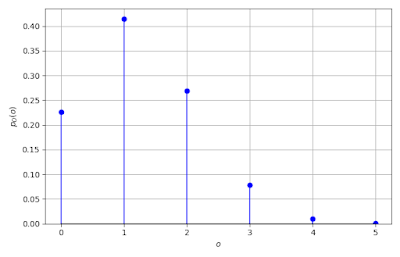Figure 7: Probability mass function of the number of outbreaks of newly infected cities immediately after an epidemic card

## Further avenues for exploration

We started off with a relatively simple question with a relatively simple answer, that the probability of drawing an epidemic card on any particular turn is 0.2, but ended up looking at quite a bit more. We ended up with both an unexpected question and answer, that it is more likely than not that there is at least one outbreak that players cannot avoid.  (Cannot avoid without special abilities or event cards, that is.)  Before getting into the conditional case, I had thought that autocorrelation and power spectral density would be useful concepts, but they ended up not really fitting in because of the finite, and rather small, number of turns in Pandemic. We could go on to compute the joint distribution of $Y_k$, but it wasn't necessary for what we were interested in, and with so many variables it's not immediately apparent how to visualize. An interesting question I'll leave unanswered but is perhaps the next in a sequence to tackle is this: what is the distribution of the total number of cards drawn from the infection deck?